# This is lab information using copper plates and an electric field. The M is the mass...

This is lab information using copper plates and an electric field. The M is the mass of the copper. The I is the amps that ran through after an hour.

MInitial (g): 19.5273 g

MFinal (g): 19.1607 g

Mass Difference (g): .3666

I (A): .32

t (s): 3600 s (1 hour)

Find the elementary charge e (C/electrons)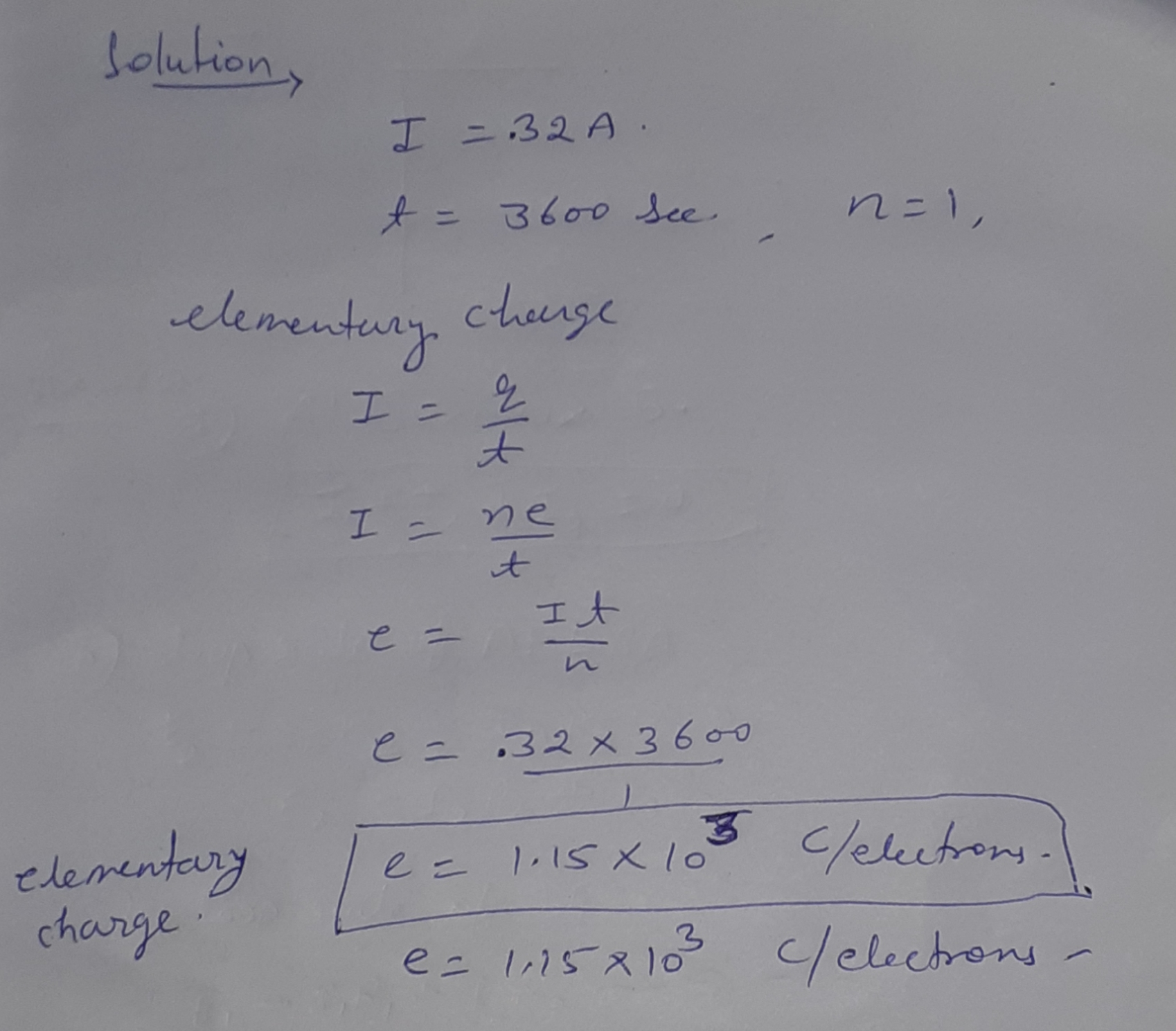##### Add Answer of: This is lab information using copper plates and an electric field. The M is the mass...
Similar Homework Help Questions
• ### The Elementary Electric Charge Data: Minitial (g): 19.527g Mfinal (g): 19.161g AM(g): 0.366g I(A): 0.320A t(s):...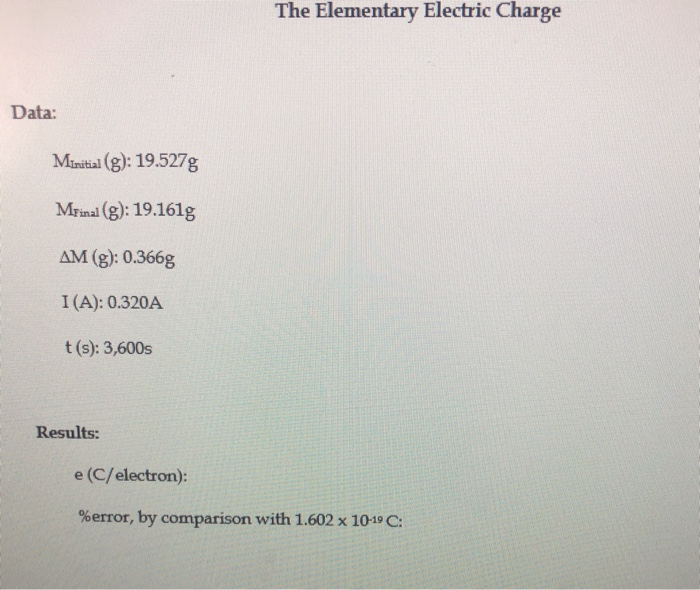The Elementary Electric Charge Data: Minitial (g): 19.527g Mfinal (g): 19.161g AM(g): 0.366g I(A): 0.320A t(s): 3,600s Results: e (C/electron): %error, by comparison with 1.602 x 10-19 C:

• ### Finding the charge to mass (e/m) ratio of the electron Often in physics, we look for ways to find fundamental physical...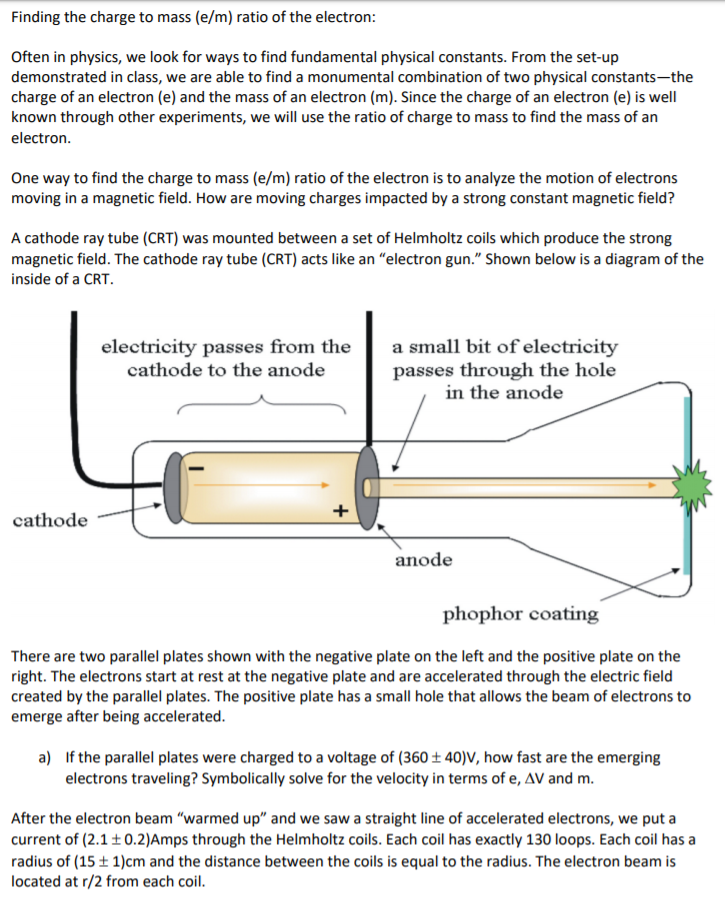Finding the charge to mass (e/m) ratio of the electron Often in physics, we look for ways to find fundamental physical constants. From the set-up demonstrated in class, we are able to find a monumental combination of two physical constants-the charge of an electron (e) and the mass of an electron (m). Since the charge of an electron (e) is well known through other experiments, we will use the ratio of charge to mass to find the mass of an...

• ### Finding the charge to mass (e/m) ratio of the electron Often in physics, we look for ways to find fundamental physical...Finding the charge to mass (e/m) ratio of the electron Often in physics, we look for ways to find fundamental physical constants. From the set-up demonstrated in class, we are able to find a monumental combination of two physical constants-the charge of an electron (e) and the mass of an electron (m). Since the charge of an electron (e) is well known through other experiments, we will use the ratio of charge to mass to find the mass of an...

• ### The ion gun of a mass spectrometer uses the electric field between charged metal plates to...

The ion gun of a mass spectrometer uses the electric field between charged metal plates to accelerate H+ ions (charge +e, mass 1.67 × 10−27 kg) from rest at the first plate to a velocity of 1.00 × 106 m s−1 at the second plate. If the higher potential plate is at +400 V find the potential of the other plate?

• ### Electric field on conducting plates effects on point charge

This is the longest question ever, so I apoligize, but any help with any part of it would be greatly appreciated. Here goes: A large conducting plate of area equal to4.0m^2 has an evenly distributed surface charge of +3.4 * 10^-6 on it's surface. A point charge of mass equal to 5.3 * 10^-6 kg and carrying a charge of 1.2 nC is held10 cm above the center of the plate. From this information determine the following:1. The electric field...

• ### tension and electric field problem

A small sphere of charge q = +69 µC and mass m = 5.0 g is attached to a lightstring and placed in a uniform electric field that makes an angle ? = 32° withthe horizontal. The opposite end of the string is attached to a wall and theh i i t ti sphere is in static equilibrium when the string is horizontal as in the figureshown below.(a) Construct a free body diagram for the sphere.(b) Find the magnitude of...

• ### A uniform electric field exists in the region between two oppositely charged parallel plates 1.50 apart....

A uniform electric field exists in the region between two oppositely charged parallel plates 1.50 apart. A proton is released from rest at the surface of the positively charged plate and strikes the surface of the opposite plate in a time interval 1.41×10−6 . A) Find the magnitude of the electric field. Use 1.60×10−19 for the magnitude of the charge on an electron and 1.67×10−27 for the mass of a proton. ------ N/C B)Find the speed of the proton at...

• ### EL These two parallel metal plates of length L-1.0 m have a uniform electric field of...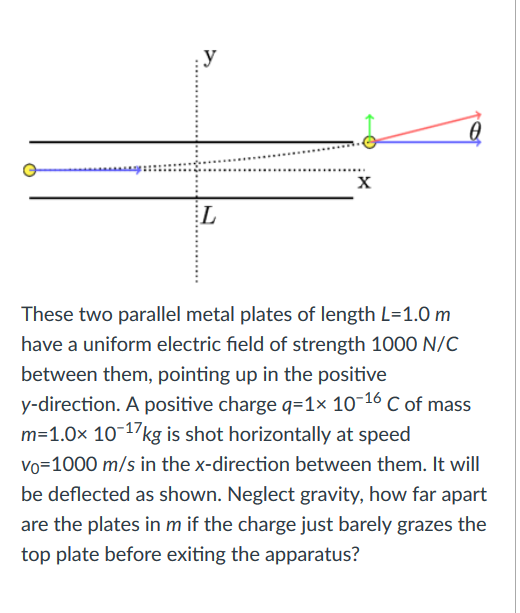EL These two parallel metal plates of length L-1.0 m have a uniform electric field of strength 1000 N/C between them, pointing up in the positive y-direction. A positive charge q-1x 1016 C of mass m-1.0x 10-17kg is shot horizontally at speed Vo-1000 m/s in the x-direction between them. It will be deflected as shown. Neglect gravity, how far apart are the plates in m if the charge just barely grazes the top plate before exiting the apparatus?

• ### A uniform electric field exists in the region between two oppositely charged parallel plates 1.56 cm...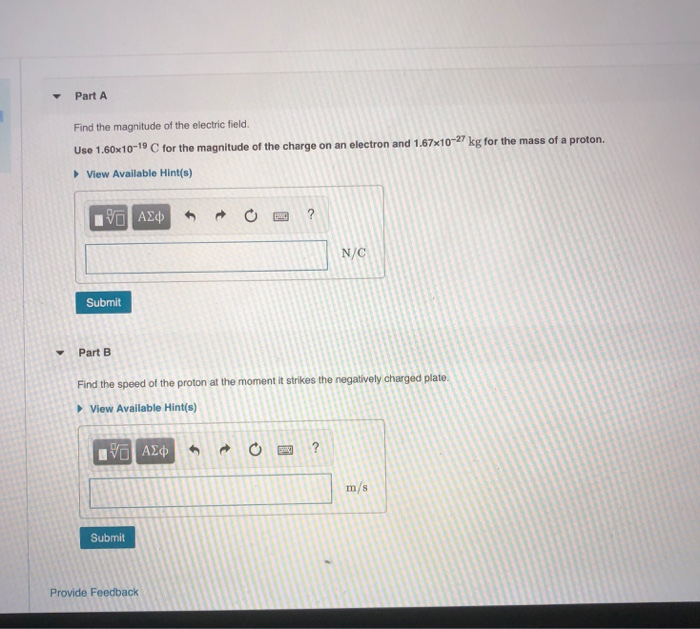A uniform electric field exists in the region between two oppositely charged parallel plates 1.56 cm apart. A proton is released from rest at the surface of the positively charged plate and strikes the surface of the opposite plate in a time interval 1.54×10^−6 s. Part A Find the magnitude of the electric field. Use 1.60x10-19 C for the magnitude of the charge on an electron and 1.67x10-27 kg for the mass of a proton View Available Hint(s) N/C Submit...

• ### A particle of rest mass mo and charge q is accelerated from rest by a uniform (in the lab frame) electric field Ei. What are the velocity and position of the particle (as a function of time) a. in th...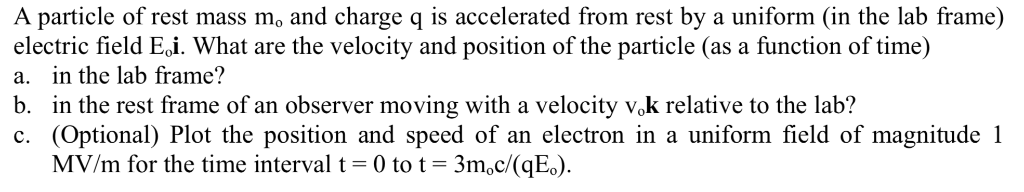A particle of rest mass mo and charge q is accelerated from rest by a uniform (in the lab frame) electric field Ei. What are the velocity and position of the particle (as a function of time) a. in the lab frame? b. in the rest frame of an observer moving with a velocity vok relative to the lab? c. (Optional) Plot the position and speed of an electron in a uniform field of magnitude 1 MV/m for the time...

Free Homework App